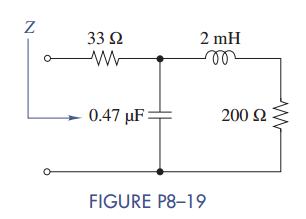Create an Account

Home / Questions / Find the equivalent impedance Z in Figure P8–19 when ω = 50 krad/s. What two elements (R, L,...

Find the equivalent impedance Z in Figure P8–19 when ω = 50 krad/s. What two elements (R, L,...

Find the equivalent impedance Z in Figure P8–19 when ω = 50 krad/s. What two elements (R, L, and/or C) could be used to replace the phasor circuit?Jul 27 2020 View more View LessSubscribe To Get Solution